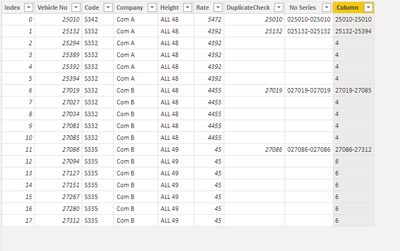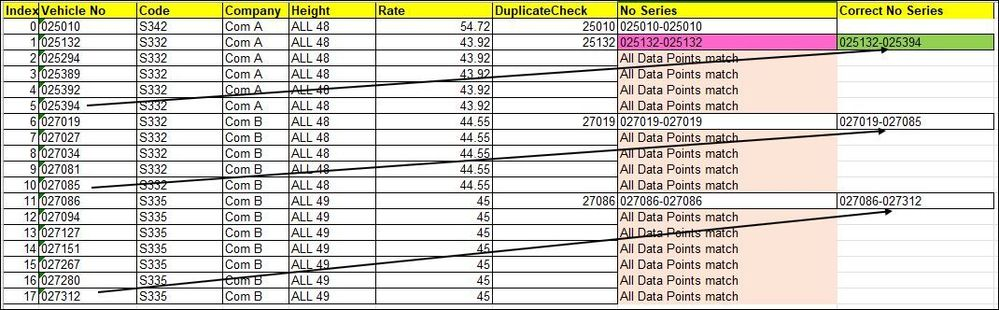cancel
Showing results for
Did you mean:

Fabric is Generally Available. Browse Fabric Presentations. Work towards your Fabric certification with the Cloud Skills Challenge.Solution Sage

## Need DAX help to generate number series based on other col value

Hi Team,

I have data like below (sample)

 Index Vehicle No Code Company Height Rate DuplicateCheck No Series 0 025010 S342 Com A ALL 48 54.72 25010 025010-025010 1 025132 S332 Com A ALL 48 43.92 25132 025132-025132 2 025294 S332 Com A ALL 48 43.92 3 025389 S332 Com A ALL 48 43.92 4 025392 S332 Com A ALL 48 43.92 5 025394 S332 Com A ALL 48 43.92 6 027019 S332 Com B ALL 48 44.55 27019 027019-027019 7 027027 S332 Com B ALL 48 44.55 8 027034 S332 Com B ALL 48 44.55 9 027081 S332 Com B ALL 48 44.55 10 027085 S332 Com B ALL 48 44.55 11 027086 S335 Com B ALL 49 45 27086 027086-027086 12 027094 S335 Com B ALL 49 45 13 027127 S335 Com B ALL 49 45 14 027151 S335 Com B ALL 49 45 15 027267 S335 Com B ALL 49 45 16 027280 S335 Com B ALL 49 45 17 027312 S335 Com B ALL 49 45

If any value change in col Code, Company, Height, Rate then new No Series should generated. But end of the No Series, should be End Vehicle No of that series,.
Now it is wrongly generated in Calcu;ated column (RED cell), require result in GREEN cellAny idea to solve this if calcuted column or measure.

1 ACCEPTED SOLUTIONCommunity Support

Hi, @PijushRoy ;

You could create a column by dax:

``````Column =
IF([Index]=
CALCULATE(MIN('Table'[Index]),ALLEXCEPT('Table','Table'[Code],'Table'[Company])),[Vehicle No]&"-"&
CALCULATE(MAX('Table'[Vehicle No]),FILTER('Table',[Code]=EARLIER('Table'[Code])&&[Company]=EARLIER('Table'[Company])
&&[Index]=CALCULATE(MAX('Table'[Index]),ALLEXCEPT('Table','Table'[Code],'Table'[Company])))))``````

or a measure

``````Measure =
IF(MAX('Table'[Index])=CALCULATE(MIN('Table'[Index]),ALLEXCEPT('Table','Table'[Code],'Table'[Company])),MAX('Table'[Vehicle No])&"-"&
CALCULATE(MAX('Table'[Vehicle No]),FILTER(ALL('Table'),[Code]=MAX('Table'[Code])&&[Company]=MAX('Table'[Company])&&[Index]=CALCULATE(MAX('Table'[Index]),ALLEXCEPT('Table','Table'[Code],'Table'[Company])))))``````

The final show:Best Regards,
Community Support Team _ Yalan Wu
If this post helps, then please consider Accept it as the solution to help the other members find it more quickly.

7 REPLIES 7Community Support

Hi, @PijushRoy ;

Try it.

``````Column =
IF([Index]=
CALCULATE(MIN('Table'[Index]),ALLEXCEPT('Table','Table'[Code],'Table'[Company])),[Vehicle No]&"-"&
CALCULATE(MAX('Table'[Vehicle No]),FILTER('Table',[Code]=EARLIER('Table'[Code])&&[Company]=EARLIER('Table'[Company])
&&[Index]=CALCULATE(MAX('Table'[Index]),ALLEXCEPT('Table','Table'[Code],'Table'[Company])))),FORMAT( CALCULATE(COUNT('Table'[Vehicle No]),ALLEXCEPT('Table','Table'[Code],'Table'[Company]))-1,"0"))``````

The final show:Best Regards,
Community Support Team _ Yalan Wu
If this post helps, then please consider Accept it as the solution to help the other members find it more quickly.Solution Sage

Hi @v-yalanwu-msft ,
I am expecting, I have created new ticket for this
https://community.powerbi.com/t5/Desktop/Counts-no-of-rows-in-calculated-column-with-blank-rows-by-D...Community Support

Hi, @PijushRoy ;

You could create a column by dax:

``````Column =
IF([Index]=
CALCULATE(MIN('Table'[Index]),ALLEXCEPT('Table','Table'[Code],'Table'[Company])),[Vehicle No]&"-"&
CALCULATE(MAX('Table'[Vehicle No]),FILTER('Table',[Code]=EARLIER('Table'[Code])&&[Company]=EARLIER('Table'[Company])
&&[Index]=CALCULATE(MAX('Table'[Index]),ALLEXCEPT('Table','Table'[Code],'Table'[Company])))))``````

or a measure

``````Measure =
IF(MAX('Table'[Index])=CALCULATE(MIN('Table'[Index]),ALLEXCEPT('Table','Table'[Code],'Table'[Company])),MAX('Table'[Vehicle No])&"-"&
CALCULATE(MAX('Table'[Vehicle No]),FILTER(ALL('Table'),[Code]=MAX('Table'[Code])&&[Company]=MAX('Table'[Company])&&[Index]=CALCULATE(MAX('Table'[Index]),ALLEXCEPT('Table','Table'[Code],'Table'[Company])))))``````

The final show:Best Regards,
Community Support Team _ Yalan Wu
If this post helps, then please consider Accept it as the solution to help the other members find it more quickly.Solution Sage

Hi @v-yalanwu-msft
like
25132-25394 for below 4 blank cell
or
Count of 25132-25394 i.e. 5Memorable Member

Hi @PijushRoy ,

Try this:

``````FindCorrectDupes =

VAR _GroupRecords = CALCULATETABLE(ALLSELECTED('YOUR_TABLE'), 'YOUR_TABLE'[Code] = EARLIER([Code]) && 'YOUR_TABLE'[Company] = EARLIER([Company]) && 'YOUR_TABLE'[Height] = EARLIER([Height]) && 'YOUR_TABLE'[Rate] = EARLIER([Rate]))
VAR _MaxID = CALCULATE(MAX('YOUR_TABLE'[Index]), FILTER('YOUR_TABLE', 'YOUR_TABLE'[Code] = EARLIER([Code]) && 'YOUR_TABLE'[Company] = EARLIER([Company]) && 'YOUR_TABLE'[Height] = EARLIER([Height]) && 'YOUR_TABLE'[Rate] = EARLIER([Rate])))

VAR _Result = IF(
LEN([No Series]) > 0 && COUNTROWS(_GroupRecords) > 1,
VAR _CurrVehicle = [Vehicle No]
VAR _MaxVehicle = LOOKUPVALUE('YOUR_TABLE'[Vehicle No], [Index], _MaxID)
RETURN
_CurrVehicle & "-" & _MaxVehicle
)

RETURN

_Result``````Solution Sage

Hi @hnguy71
Problem I faced the first row I got blank and trying to fix this, where got another solution from @v-yalanwu-msft and works perfectly after makes some changes.

Thanks again for your prompt and useful DAXSolution Sage

Hi,
For solution, it is ok to replace the blank cell with any value in Duplicate Check colAnnouncements#### Power BI Monthly Update - November 2023

Check out the November 2023 Power BI update to learn about new features.#### Fabric Community News unified experience

Read the latest Fabric Community announcements, including updates on Power BI, Synapse, Data Factory and Data Activator.#### The largest Power BI and Fabric virtual conference

130+ sessions, 130+ speakers, Product managers, MVPs, and experts. All about Power BI and Fabric. Attend online or watch the recordings.Top Solution Authors
Top Kudoed Authors
Users online (4,636)